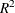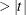# The PDLREG Procedure

### Printed Output

The PDLREG procedure prints the following items:

1. the name of the dependent variable

2. the ordinary least squares (OLS) estimates

3. the estimates of autocorrelations and of the autocovariance, and if line size permits, a graph of the autocorrelation at each lag. The autocorrelation for lag 0 is 1. These items are printed if you specify the NLAG= option.

4. the partial autocorrelations if the PARTIAL and NLAG= options are specified. The first partial autocorrelation is the autocorrelation for lag 1.

5. the preliminary mean square error, which results from solving the Yule-Walker equations if you specify the NLAG= option

6. the estimates of the autoregressive parameters, their standard errors, and the ratios of estimates to standard errors (t ) if you specify the NLAG= option

7. the statistics of fit for the final model if you specify the NLAG= option. These include the error sum of squares (SSE), the degrees of freedom for error (DFE), the mean square error (MSE), the root mean square error (Root MSE), the mean absolute error (MAE), the mean absolute percentage error (MAPE), the Schwarz information criterion (SBC), the Akaike’s information criterion (AIC), Akaike’s information criterion corrected(AICC), the regression(Regress R-Square), the total(Total R-Square), and the Durbin-Watson statistic (Durbin-Watson). See Chapter 8: The AUTOREG Procedure, for details of the regressionand the total.

8. the parameter estimates for the structural model (B), a standard error estimate, the ratio of estimate to standard error (t), and an approximation to the significance probability for the parameter being 0 ("Approx Pr")

9. a plot of the lag distribution (estimate of lag distribution)

10. the covariance matrix of the parameter estimates if the COVB option is specified